# Analysis and Design of Algorithms

Jul7 17, 2019

• Chapter 27 of Introduction to Algorithms (3rd Edition), Thomas H. Cormen, Charles E. Leiserson, Ronald L. Rivest, and Clifford Stein.

• Most algorithms in the book are serial algorithms
• Run on a uniprocessor computer
• Execute one instruction at a time
• In this chapter, we will see parallel algorithms
• Run on a multiprocessor computer
• Allows multiple instructions to execute concurrently
• Explore an elegant model: dynamic multithreaded algorithms

• Parallel computers
• Computers with multiple processing units
• Very common, with different prices and performance
• Relatively inexpensive:
• Desktop and laptop chip multiprocessors
• Single multi-core integrated circuit chip
• Houses multiple processing cores
• Each core is a full processor that can access a common memory
• Intermediate price/performance:
• Clusters built from individual computers
• Can be simple PC-class machines with a dedicated network interconnecting them
• Highest priced machines:
• Supercomputers
• Combination of custom architectures and custom networks
• Designed to deliver the highest performance (instructions per second, i.e. flops)

• Multiprocessor computers have been around for a while
• There is not a single model for parallel computing with wide acceptance
• Vendors have not agreed on a single parallel architectural model
• Some feature shared memory
• Others employ distributed memory
• With multi-core technology, new laptops and desktops are a shared-memory parallel computer
• Trend appears to be toward shared-memory multiprocessing

• Common means of programming chip multiprocessors (and other shared memory parallel computers)
• Provides a software abstraction of virtual processors or threads sharing common memory
• Each thread mantains an associated program counter
• Can execute code independently of the other threads
• Switches it out when another thread needs to run

• The OS allows the user to create and destroy threads
• But these operations are very slow
• Thus, in most apps, threads persist for the duration of a computation
• That’s why they are called static
• Programming when using static threads
• It’s difficult and error-prone
• Programmer requires complex communication protocols
• To implement a scheduler to load-balance the work
• Concurrency platforms were created to deal with this complexity
• Provide a SW layer to coordinate, schedule, and manage the parallel-computing resources

• Important concurrency platform
• The model used in this lecture
• Allows programmers specify parallelism in applications without worrying about
• Communication protocols
• Other…

• Concurrency platform contains a scheduler
• Simplifies the work of the programmer
• Functionality of dynamic-multithreading provides 2 features
• Nested parallelism
• Allows a subroutne to be spawned to allow the caller to proceed while the spawned subroutine is computing the result
• Parallel loops
• It is like an ordinary for loop but the iterations of the loop can execute concurrently
• Key aspect of this model
• Programmer specifies only the logical parallelism within a computation
• Threads in the platform schedule and load-balance the computation among themselves

• It’s a simple extension of the serial programming model
• We just add three keywords to our pseudocode:
• parallel
• spawn
• sync
• If we remove the keywords, we get the serial pseudocode for the same problem
• The serialization of the multithreaded algorithm

• Provides a theoretically clean way to quantify parallelism
• Based on notions of work and span

• We use recurrences also for multithreaded algorithms of this type

• Model shows how parallel-computing practice evolves
• Growing number of concurrency platforms
• Support a variant of dynamic multithreading
• Cilk
• Cilk++
• OpenMP

# The Basics of Dynamic Multithreading

• Example: Computing Fibonacci numbers recursively
• Fibonacci numbers defined by recurrence
• $$F_0 = 0$$
• $$F_1 = 1$$
• $$F_i = F_{i-1} + F_{i-2}$$ for $$i \geq 2$$.

# The Basics of Dynamic Multithreading

• A simple, recursive, serial algorithm to compute the $$n$$th Fibonnaci number: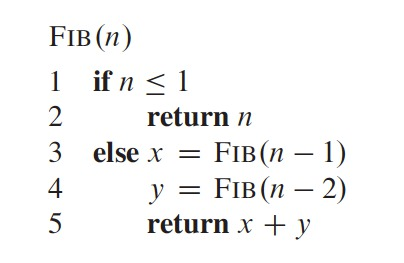# The Basics of Dynamic Multithreading

• This algorithm repeats too much work (it doesn’t memoize)
• The recursive tree of the call for Fib(6)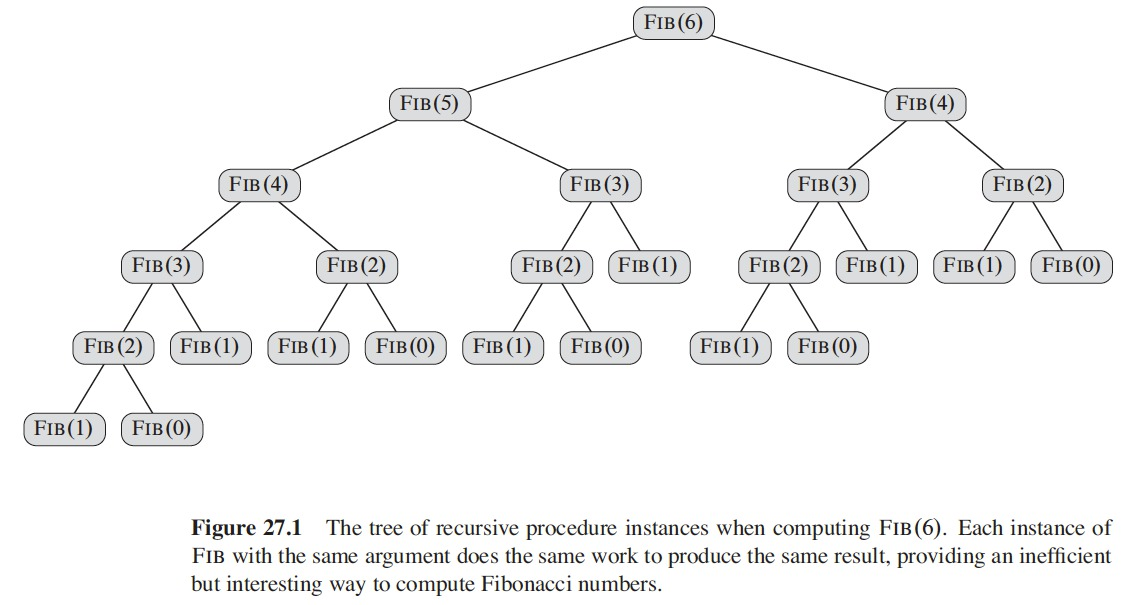# The Basics of Dynamic Multithreading

• The recurrence for the Fibonacci algorithm
• $$T(n) = T(n-1) + T(n-2) + \Theta(1)$$.
• Solution: $$T(n) = \Theta(F_n)$$
• $$F_n$$ grows exponentially in $$n$$
• This is slow…, but it is illustrative for this purpose
• The analysis of multithreaded algorithms

# The Basics of Dynamic Multithreading

• The 2 recursive calls in lines 3 and 4 are independent of each other
• $$FIB(n-1)$$ and $$FIB(n-2)$$
• They can be called in either order
• The computation of one of them doesn’t affect the other
• They can be run in parallel
• Augment the pseudocode to indicate parallelism
• spawn
• sync

# The Basics of Dynamic Multithreading

• Pseudocode rewriten to use dynamic multithreading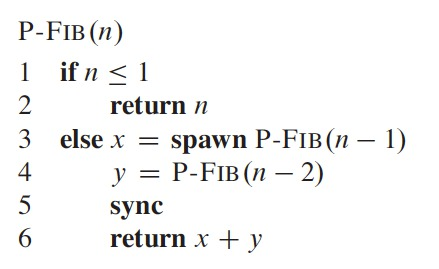# The Basics of Dynamic Multithreading

• Deleting the concurrency keywords (spawn and sync) to P-FIB
• Produces the FIB pseudocode
• This is known as serialization
• Serialization of a multithreaded algorithm
• The serial algorithm resulting when deleting the multithreading keywords
• spawn, sync, and parallel

# The Basics of Dynamic Multithreading

• Nested parallelism
• When the spawn keyword precedes a procedure call
• i.e. As in line 3
• When we make a call with spawn
• Similar to a fork in unix
• The parent may continue execution in parallel with the spawned subroutine, the child
• It doesn’t wait for the child to complete in order to continue with the execution
• The child computes $$P-FIB(n-1)$$
• The parent computes $$P-FIB(n-2)$$ in parallel with the spawned child
• Because $$P-FIB$$ is recursive, the 2 subroutine calls themselves create nested parallelism
• And also their children
• Creates a tree of subcomputations, all executing in parallel

# The Basics of Dynamic Multithreading

• The concurrency keywords express the logical parallelism of the computation
• Indicates which parts of code may proceed in parallel
• At runtime, the scheduler determines which subcomputations run concurrently
• Assigns available processors

# The Basics of Dynamic Multithreading

• The sync keyword
• The parent procedures executes the sync statement
• In order to safely use the results returned by its children
• Sync indicates that the parent procedure must wait for all its spawned children to complete, before proceeding
• In $$P-FIB$$ the procedure calls sync before the return statement

# A Model for Multithreaded Execution

• A multithreaded computation can be seen as
• A set of runtime instructions executed by a processor to complete a multithreaded program
• A multithreaded computation can be modeled as a directed acyclic graph $$G = (V, E)$$, called a computation dag

# A Model for Multithreaded Execution

• A Computation dag for the $$P-FIB$$ procedure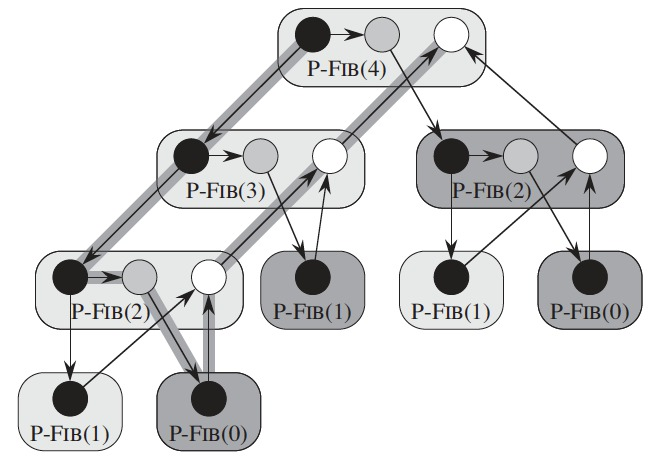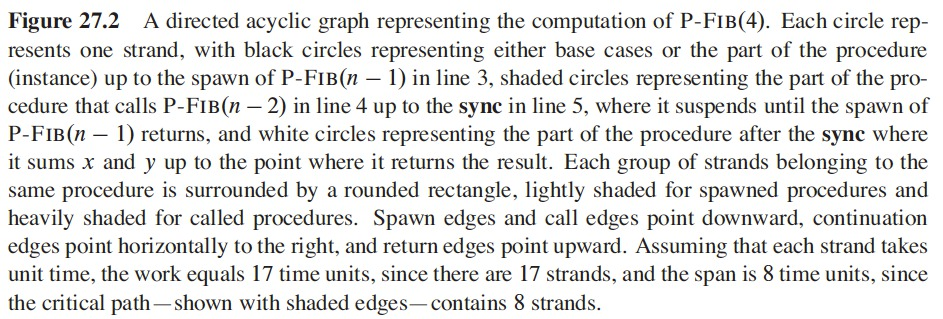# A Model for Multithreaded Execution

• Computation dag
• Vertices $$V$$ are instructions
• Edges $$E$$ represent dependencies between instructions
• $$(u,v) \in E$$ means instruction $$u$$ must execute before instruction $$v$$
• If a chain of instructions has no call to a parallel control keyword, we group them into a single strand
• A strand represents one or more instructions
• Instructions involving parallel control are not included in strands but appear in the structure of the dag
• If a strand has 2 successors, one of them must have been spawned
• A strand with multiple predecessors means that they were joined with a sync statement
• In general:
• $$V$$ represents the strands
• $$E$$ represents the dependencies between strands induced by parallel control

# A Model for Multithreaded Execution

• Computation dag
• If $$G$$ has a directed path from strand $$u$$ to strand $$v$$
• The two strands are (logically) in series
• Otherwise, strands $$u$$ and $$v$$ are (logically) in parallel
• We picture a multithreaded computation with a dag of strands embedded in a tree of procedure instances

# A Model for Multithreaded Execution

• Tree of procedure instances for $$P-FIB(6)$$, no detailed structure of strands is shown# A Model for Multithreaded Execution

• Zoom of a section of the previous tree, shows the strands of each procedure
• Directed edges connecting strands run either
• Within a procedure
• Along the procedure tree# A Model for Multithreaded Execution

• Edges of a computation dag indicate a type of dependency between strands
• Continuation edge $$(u, u')$$, drawn horizontally
• Connects a strand $$u$$ to its successor $$u'$$ within the same procedure instance
• Spawn edge $$(u, v)$$, points downward in the figure
• When a strand $$u$$ spawns a strand $$v$$
• Call edge, also point downward
• Represent normal procedure calls
• Return edge $$(u, x)$$, points upward
• When a strand $$u$$ returns to its calling procedure
• $$x$$ is the strand immediately following the next sync
• Initial strand, starts a computation
• Final strand, ends a computation
• Difference between a spawn edge and a call edge
• Strand $$u$$ spawning strand $$v$$
• Induces a horizontal continuation edge from $$u$$ to the strand $$u'$$ following $$u$$ in its procedure
• $$u'$$ is free to execute at the same time as $$v$$
• $$u$$ calling $$v$$
• Doesn’t induce such an edge

# A Model for Multithreaded Execution

• We assume our multithreaded algorithms execute on an ideal parallel computer
• Set of processors
• Sequentially consistent shared memory
• Sequentially consistent
• Memory preserves the individual orders in which each processor executes its instructions
• Memory behaves as if instructions were interleaved to produce a linear order to preserve the partial order of the computation dag
• Order might differ from one run to the other (depending on scheduling) but is consistent with the computation dag

# A Model for Multithreaded Execution

• Performance assumptions
• Each processor has equal computing power
• Ignores the cost of scheduling
• With sufficient parallelism the overhead of scheduling is minimal in practice

# A Model for Multithreaded Execution

• Performance measures
• Work
• Total time to execute the entire computation on one processor
• The sum of the times by each of the strands
• In a computation dag in which each strand takes a unit of time
• The work is the number of vertices in the dag
• Span
• The longest time to execute the strands along any path in the dag
• If each strand takes a unit time: the number of vertices on a longest or critical path in the dag

# A Model for Multithreaded Execution

• The actual running time of a multithreaded depends on
• Work and span
• How many processors are available
• How the scheduler allocates strands to processors
• We denote running time on $$P$$ processors using subscript $$P$$
• Running time of an algorithm on $$P$$ processors: $$T_P$$
• Work is $$T_1$$, in a single processor
• Span is $$T_{\infty}$$, corresponds to running each strand on its own processor
• As if having $$\infty$$ number of processors
• Work and span are lower bounds on running time $$T_P$$ of a multithreaded computation on $$P$$ processors

# A Model for Multithreaded Execution

• Work law: $$T_P \geq T_1/P$$
• In ideal parallel computer with $$P$$ processors can do at most $$P$$ units of work
• In $$T_P$$ time it can perform at most $$PT_P$$ work, and total work is $$T_1$$
• We have $$PT_P \geq T_1$$, we divide by $$P$$ and get the work law

# A Model for Multithreaded Execution

• Span law: $$T_P \geq T_{\infty}$$
• A $$P$$-processor ideal computer can’t run faster than a machine with unlimited number of processors
• Then, a machine with unlimited processors emulates a $$P$$-processor machine by using $$P$$ of its processors

# A Model for Multithreaded Execution

• Speedup of a computation on $$P$$ processors is the ratio $$T_1/T_P$$
• How many times faster is the computation with $$P$$ processors than with 1 processor
• By work law: $$T_P \geq T_1/P$$, then $$T_1/T_P \leq P$$
• Then speedup on $$P$$ processors can be at most $$P$$
• Linear speedup
• The speed up is linear in the number of processors
• $$T_1/T_P = \Theta(P)$$
• Perfect linear speedup
• $$T_1/T_P = P$$
• Parallelism of the multithreaded computation
• The ratio of the work to the span: $$T_1/T_{\infty}$$
• 3 perspectives of parallelism
• As a ratio: average amount of work (in parallel) for each step along the critical path
• As an upper bound: maximum possible speedup that can be achieved on any number of processors
• As a limit: on the possibility of attaining perfect linear speedup

# A Model for Multithreaded Execution

• Example: computation of $$P-FIB(4)$$, assume each strand takes unit time
• Work is $$T_1 = 17$$
• Span is $$T_{\infty} = 8$$
• Parallelism is $$T_1/T_{\infty} = 17/8 = 2.125$$
• This means that achieving more than double the speedup is impossible (for the specific example of $$P-FIB(4)$$)
• No matter how many processors we use# A Model for Multithreaded Execution

• Parallel slackness of a multithreaded computation
• Executed on an ideal parallel computer with $$P$$ processors
• $$(T_1/T_{\infty})/P = T_1/(PT_{\infty})$$
• Factor by which parallelism of computation exceeds number of processors in the machine

# Scheduling

• Require efficient scheduling
• No way to specify which strand executes on which processor
• Rely on the concurrency platform’s scheduler
• How it works
• Scheduler maps strands to static threads
• OS schedules threads to the processors
• We assume that scheduler directly maps strands to processors

# Scheduling

• We assume an on-line centralized scheduler
• On-line: doesn’t have knowledge in advance about when strands will be spawned or when they will complete
• Centralized: knows global state of the computation at any given time
• Greedy schedulers
• Assign as many strands to processors as possible in each time step
• Complete step: There are at least $$P$$ strands ready to execute during a time step
• Incomplete step: There are less than $$P$$ strands ready to execute

# Scheduling

• Theorem 27.1
• On an ideal parallel computer with $$P$$ processors, a greedy scheduler executes a multithreaded computation with work $$T_1$$ and span $$T_{\infty}$$ in time
• $$T_P \leq T_1/P + T_{\infty}$$

# Scheduling

• Corollary 27.2
• The running time $$T_P$$ of any multithreaded computation scheduled by a greedy scheduler on an ideal parallel computer with $$P$$ processors is within a factor of 2 of optimal

# Scheduling

• Corollary 27.3
• Let $$T_P$$ be the running time of a multithreaded computation produced by a greedy scheduler on an ideal parallel computer with $$P$$ processors, and let $$T_1$$ and $$T_{\infty}$$ be the work and span of the computation, respectively. Then, if $$P << T_1/T_{\infty}$$, we have $$T_P \approx T_1/P$$, or equivalently, a speedup of approximately $$P$$.

• Analizing the work
• Straightforward (relatively)
• The running time of an ordinary serial algorithm: the serialization of the multithreaded algorithm
• Analyzing the span
• More interesting/complicated
• For 2 computations in series, their spans add to form the span of their composition
• For 2 computations in parallel, the span of their composition is the maximum of the spans of the 2 subcomputations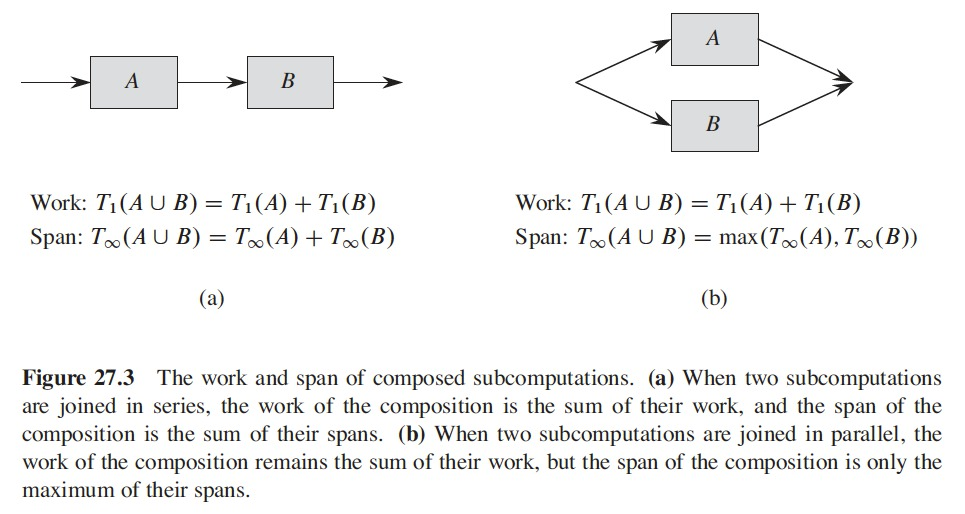• When analyzing $$P-FIB(n)$$
• The spawned call to $$P-FIB(n-1)$$ in line 3 runs in parallel with call to $$P-FIB(n-2)$$ in line 4
• We express the span of $$P-FIB(n)$$ as a recurrence
• $$T_{\infty}(n) = max(T_{\infty}(n-1), T_{\infty}(n-2)) + \Theta(1)$$
• $$= T_{\infty}(n-1) + \Theta(1)$$
• With solution: $$T_{\infty}(n) = \Theta(n)$$
• The parallelism of $$P-FIB(n)$$ is $$T_1(n)/T_{\infty}(n) = \Theta(\Phi^n/n)$$

# Parallel loops

• Many algorithms have loops for which iterations can run in parallel
• We can parallelize those loops using spawn and sync
• It’s more convenient to specify that the iterations of the loop can run concurrently
• We use the parallel concurrency keyword
• Precedes the for keyword in a for loop statement

# Parallel loops

• Example: multiplying an $$n \times n$$ matrix $$A = (a_{ij})$$ by an $$n$$-vector $$x = (x_j)$$
• $$y_i = \sum\limits_{j=1}^n a_{ij}x_j$$
• for $$i = 1, 2, \dots, n$$
• We can perform all the entries of $$y$$ in parallel as follows: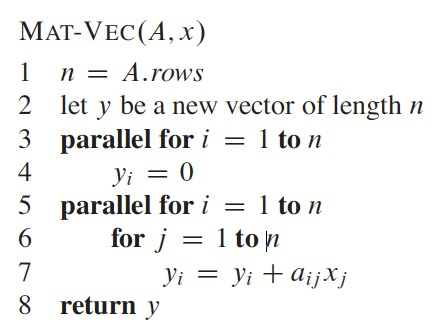# Parallel loops

• The parallel for keywords indicate the iterations of loops may run concurrently
• A compiler can implement each parallel loop as a divide and conquer routine using nested parallelism
• A call to $$MAT-VEC-MAIN-LOOP(A, x, y, n, 1, n)$$